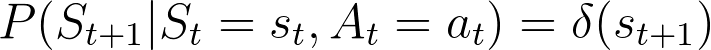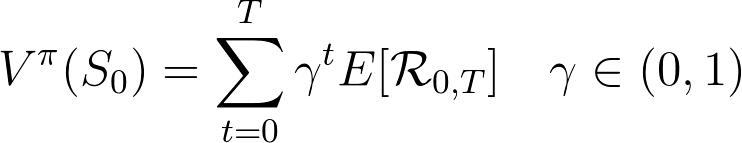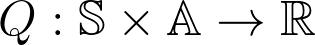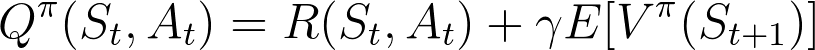# In 1 min : Q-Learning

TL;DR

Q-Learning is a Model Free Policy Learning Algo, it means it does not focus on learning the State Transition Function and the Reward Function but it tries to learn the Q-Function directly by trial-and-error with the environment

Preliminaries

Let’s start by assuming Deterministic Evolution Function and Reward Function (it won’t be hard to generalize to the case of Probabilistic Evolution)

A Policy is just a function which associates States and Actions, let’s assume it also is deterministic

Selecting a State and a Policy automatically defines a full Trajectory in the State — Action Space

Applying the Reward it is possible to compute the associates Reward Succession

The Value Function just performs a Temporal Marginalization using a Discount Factor to associate a Scalar Value to a State — Policy PairValue Function as Discounted Temporal Marginalization of Reward Succession. In general the Reward Succession could be composed of Random Variables, hence the Expected Value

A Value can be interpreted as both the Value a specific State has for a given Policy and the Value a given Policy has in a given State

Yet an element is missing to learn a policy hence to learn to associates Actions to States: the Q-Function
The Q-Function assumes a State and a Policy but but it also takes an Action as Input and returns the Value of choosing that Action (regardless of the Policy) as immediate decision, discounting the future states using the Value of the future StateQ-Function: mapping from State — Action Space to Real Value space, used to represents RewardsQ-Function is defined considering the policy-independent choice of A_{t} while then discounting the future states with Value Function

The Policy Learning consisting of State — Action association learning can then be performed iteratively by local updates (at specific State Level) by choosing the Action with maximizes Q-Function
As the Q-Function, used to learn the policy, is also depending on the policy the learning process is a dynamic system and it’s possible to time-tag the policy properly